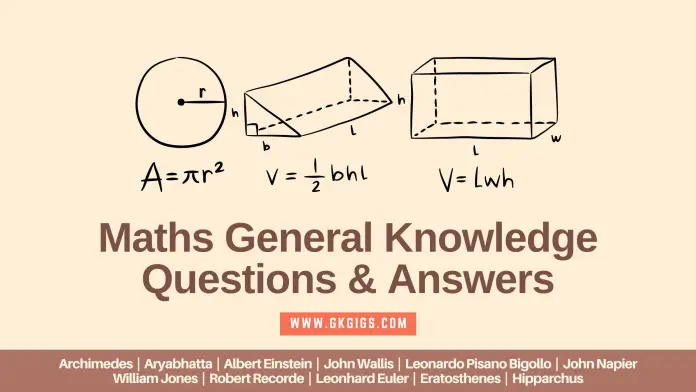# Maths GK Question And Answer (Short & Important)Last Updated: 7 January 2023

All of us use maths in our day-to-day activities without realizing it.

From purchasing something to saving your monthly pocket money to measuring quantities while cooking or selling a thing. We take the help of numbers every time, meaning we use maths to solve our problems and make our life easy.

Although we use maths we don’t know what and who invented or discovered it in the field of mathematics. This blog has all the Important Maths questions that you may find useful.

Let us look at some of the GK Questions and Answer on Maths and expand your learning.

1. Most famous Theorem of Triangles in Geometry?

2. How many Faces are there in a Pyramid?

3. Roman Number of 400?

4. Roman Number of 500?

5. Roman Number of 600?

6. Roman Number of 900?

7. Roman Number of 1000?

8. Angles greater than 90 degrees but less than 180 degrees are called?

9. How many sides are there in a Heptagon?

10. Solid shape that has four equal triangles?

11. What part of a Revolution have you turned through if you stand facing west and turn clockwise to face South?

12. Who discovered ∮ the Contour Integral sign?

13. Who discovered Existential Quantifier ∃ (there exists)?

14. Who invented ± Plus-Minus sign?

15. Father of Cryptology?

16. What is the shape of a Brick in India?

17. Where did the “Magic Square” originate?

18. Which movie is based on Srinivasa Ramanujan?

Answer: The Man Who Knew Infinity

19. Number between 50 and 60, which is a multiple of 7 and 8?

20. Who invented “∇” the Nabla symbol?

21. Who is known as Human Computer?

22. Name of the symbol φ ?

23. Name of the symbol Δ ?

24. How many Centimeters make a Decameter?

25. What is the name of the following sequences 0,1,1,2,3,5,8….?

26. Who discovered “/” Division Slash (solidus)?

27. Ashok has an equal number of one rupee, five rupees, and 10 rupees notes. If he has ₹320 with him, what will be the number of each rupee note?

28. What is the other name of the Perimeter of a Circle?

29. Who discovered the Proportionality Sign ∝ ?

30. Who discovered the partial differential sign ∂ (curly d or Jacobi’s delta)?

Related: Exponents And Powers Multiple Choice Questions (CBSE & ICSE)

31. Who discovered Partial Derivatives?

32. Who discovered Gamma-Function “Γ” ?

33. Who discovered Laplace Transform?

34. Who invented the Factorial symbol “!” ?

35. Who said the phrase “There is no permanent place in the world for ugly mathematics”?

36. Who is the Father of Analytic Geometry?

Answer: René Descartes and Pierre de Fermat

37. Who invented Parentheses ( ) ?

38. Who invented “∫” Integral sign?

39. Who discovered Matrix Notation […] ?

40. Who is the “Father of Mathematics”?

41. Name of the polygon that has 15 sides?

42. Who discovered an easy method to find all the Prime Numbers?

43. What is the average of the first 50 Natural Numbers?

44. What is an angle greater than 180 degrees but less than 360 degrees called?

45. The Smallest Perfect Number?

46. Who invented the Radical symbol √ ̅ ( square root)?

47. Mr. Harold ranked 7th from the top and 26th from the bottom, How many students are there in the class?

48. Who discovered ” ∀ ” universal quantifier (for all)?

49. A number between 30 and 50 which is a common multiple of 6 and 8. What is the number when it is added to 45?

50. Roman Number of 40?

Find Mathematics General Knowledge Questions And Answers PDF here.

51. What is the Line called which cuts a pair of Parallel lines?

52.  Who developed the Chinese Remainder Theorem?

Related: Area And Perimeter Multiple Choice Questions

53.  Who developed Descartes’ Theorem?

54. Who created the Theory of Probability?

Answer: Blaise Pascal and Pierre de Fermat

55. Who was the first person to use Algebra for solving Astronomical Problems?

56. Which equation was solved by Rafael Bombelli using Imaginary numbers?

57. Who discovered Binomial Theorem?

58. Who discovered Divergence Theorem?

59. Who discovered Taylor Series?

60. Calculate the Rate of Interest for a certain sum of money that becomes 5 times its principal Amount in 10 years?

61. Which Theorem did André-Marie Ampère discover in 1825?

62. Who developed the Analytic number theory?

63. Which problem was solved by Jean le Rond d’Alembert in 1747?

Answer: Vibrating string problem (one-dimensional wave equation)

64.  Who proved the Fundamental Theorem of Arithmetic?

Answer: Euclid (sometimes called Euclid of Alexandria)

65. Who proved that the value of π lies between 3 + 1/7 (approx. 3.1429) and 3 + 10/71 (approx. 3.1408)?

66. Which theorem did Thomas Bayes prove in 1761?

67. Who Proved that “π” Pi is Irrational?

68. Which Numerals have been modified by Arab mathematicians to form the modern Arabic Numeral system?

69. Which formulae did Al-Batani in AD 975 derive after extending the Indian concepts of sine and cosine to other trigonometrical ratios, like a tangent, secant, and their inverse functions?

Answer:70. Which rule did Shridhara (A.K.A Sridharacharya from India) give?

Answer: Volume of a sphere and the formula for solving quadratic equations

71. Who first introduced the Trigonometric Functions (sine and cosine) and methods of calculating their Approximate Numerical Values?

1.RIYA SHNDA